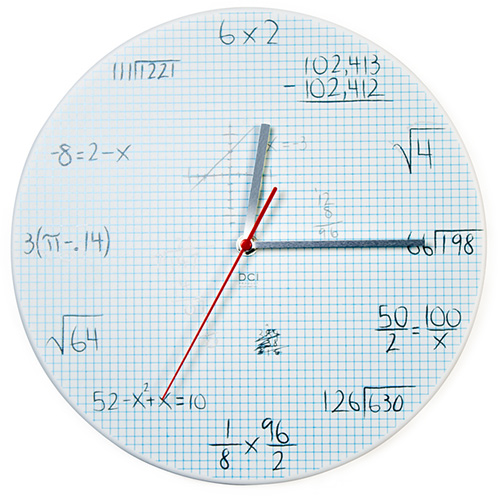# Pop Quiz Math Equations Wall ClockThis geeky clock has a series of math problems replacing the normal numbers on the clock face. If that sounds like fun to you, this Pop Quiz Math Equations Wall Clock needs to be on your wish list.  Adding to the coolness (or geekiness?), the math problems look like they are written on old school graph paper, although it is actually made out of metal.

You don’t exactly need to be a mathematics whiz to solve these problems; a knowledge of basic algebra and knowing the value of Pi to two decimal places is sufficient.

Pop Quiz Math Equations Wall Clock Features:

• Made of lightweight white metal with a graph paper look background
• Work out the equations to determine the hour
• Easy-to-hang with “keyhole” opening on the back of the clock
• Requires 1 AA Battery (not included)
• Measures 11.5″ (29 cm) diameter

The Pop Quiz Math Equations Wall Clock is available at Amazon.com. It is available to pre-order for £29.99 at Firebox.com.

#### 1 Comment

1. Sadly, this clock assumes that pi is equal to 3.14. 🙁